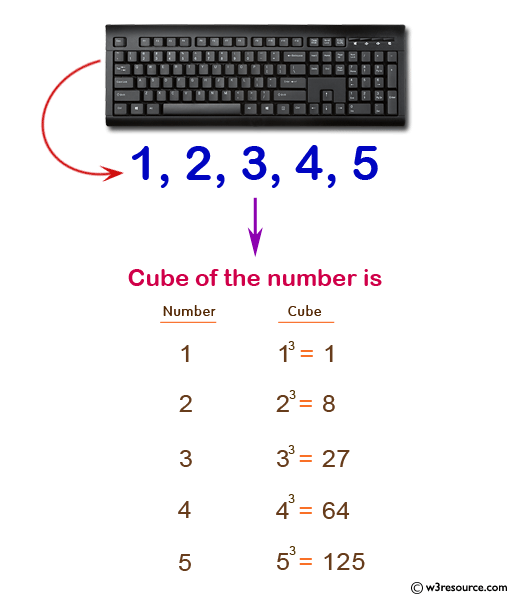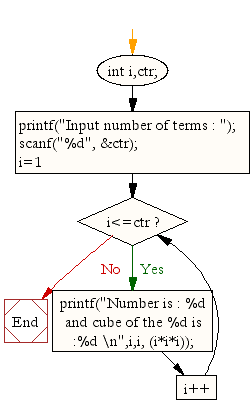﻿ C Program: Find cube of the number upto a given integer - w3resource# C Exercises: Find cube of the number upto a given integer

## C For Loop: Exercise-5 with Solution

Write a program in C to display the cube of the number upto a given integer.

Pictorial Presentation:Sample Solution:

C Code:

``````#include <stdio.h>
void main()
{
int i,ctr;
printf("Input number of terms : ");
scanf("%d", &ctr);
for(i=1;i<=ctr;i++)
{
printf("Number is : %d and cube of the %d is :%d \n",i,i, (i*i*i));
}
}
```
```

Sample Output:

```Input number of terms : 5
Number is : 1 and cube of the 1 is :1
Number is : 2 and cube of the 2 is :8
Number is : 3 and cube of the 3 is :27
Number is : 4 and cube of the 4 is :64
Number is : 5 and cube of the 5 is :125
```

Flowchart:C Programming Code Editor:

Improve this sample solution and post your code through Disqus.

What is the difficulty level of this exercise?

Test your Programming skills with w3resource's quiz.

﻿